### 网站开发

•••## 网站开发

### \$(function() { \$('label').click(function(){var aa = \$(this).attr('aa');\$('[aa="'+aa+'"]').removeAttr('class') ;\$(this).attr('class','checked');});});function check(){if(\$('#0_0').get(0).checked||\$('#0_1').get(0).checked||\$('#0_2').get(0).checked||\$('#0_3').get(0).checked){}else{swal({title:'提示',text:'请选择商品属性',type:'error'},function(){});return false;} if(\$('#1_0').get(0).checked||\$('#1_1').get(0).checked||\$('#1_2').get(0).checked||\$('#1_3').get(0).checked){}else{swal({title:'提示',text:'请选择商品属性',type:'error'},function(){});return false;} if(\$('#2_0').get(0).checked||\$('#2_1').get(0).checked||\$('#2_2').get(0).checked||\$('#2_3').get(0).checked){}else{swal({title:'提示',text:'请选择商品属性',type:'error'},function(){});return false;} }function shuaxin(){if(\$('#0_0').get(0).checked){sp0=0}; if(\$('#0_1').get(0).checked){sp0=0}; if(\$('#0_2').get(0).checked){sp0=0}; if(\$('#0_3').get(0).checked){sp0=100}; if(\$('#1_0').get(0).checked){sp1=0}; if(\$('#1_1').get(0).checked){sp1=1000}; if(\$('#1_2').get(0).checked){sp1=2000}; if(\$('#1_3').get(0).checked){sp1=3000}; if(\$('#2_0').get(0).checked){sp2=100}; if(\$('#2_1').get(0).checked){sp2=0}; if(\$('#2_2').get(0).checked){sp2=100}; if(\$('#2_3').get(0).checked){sp2=200}; \$('#price').html((3000+sp0+sp1+sp2).toFixed(2)); \$('#P_price').val(3000+sp0+sp1+sp2)}价格：3000 元运营商 移动 联通 电信 全网通 容量 16G 32G 64G 128G 颜色 银色 灰色 土豪金 玫瑰金 购买数量：（库存：件）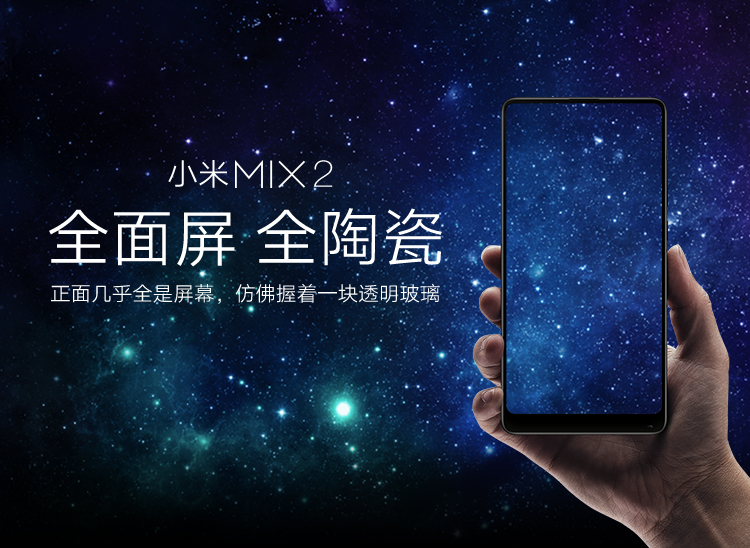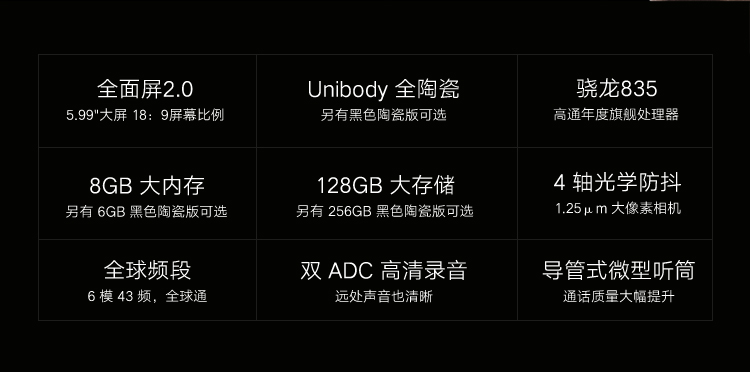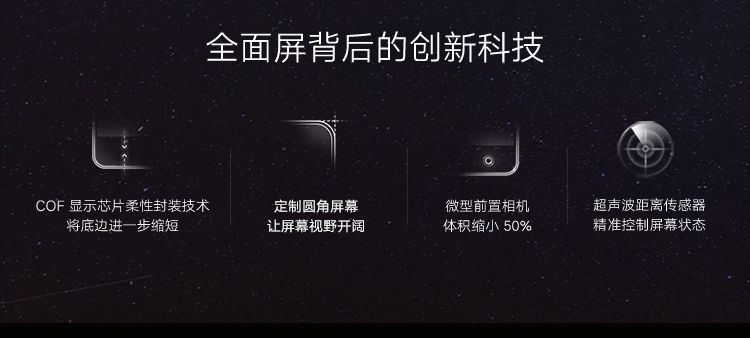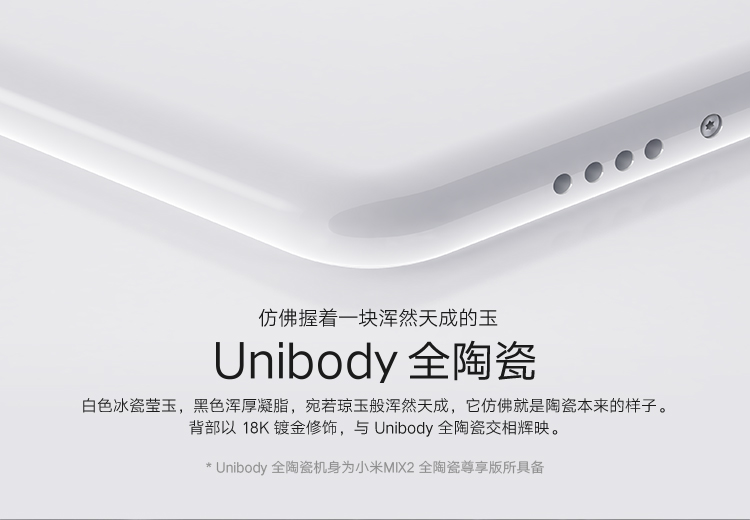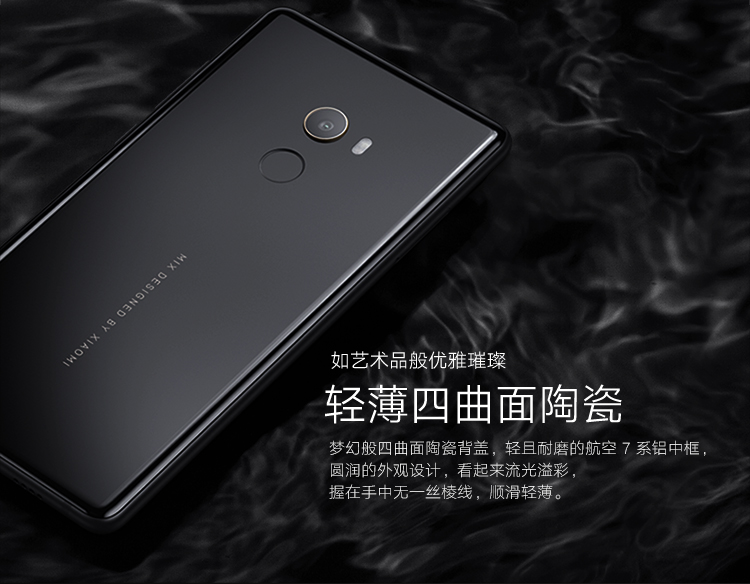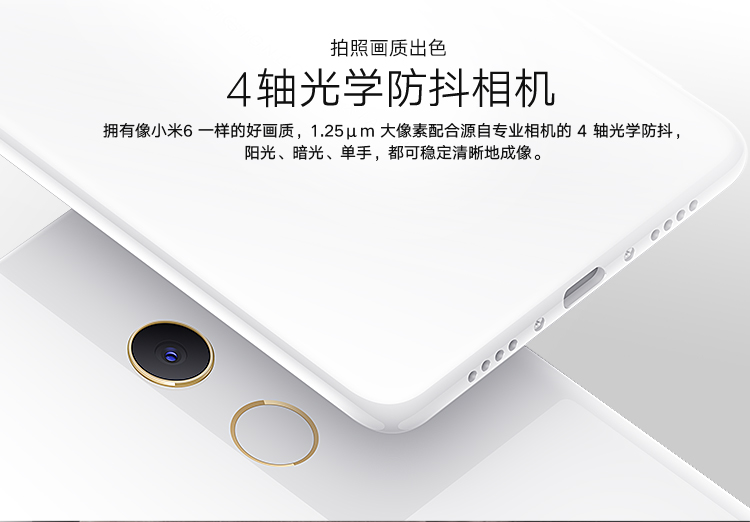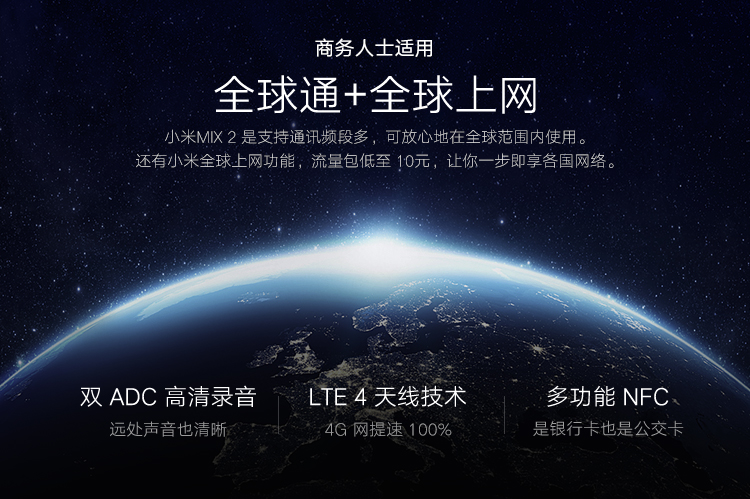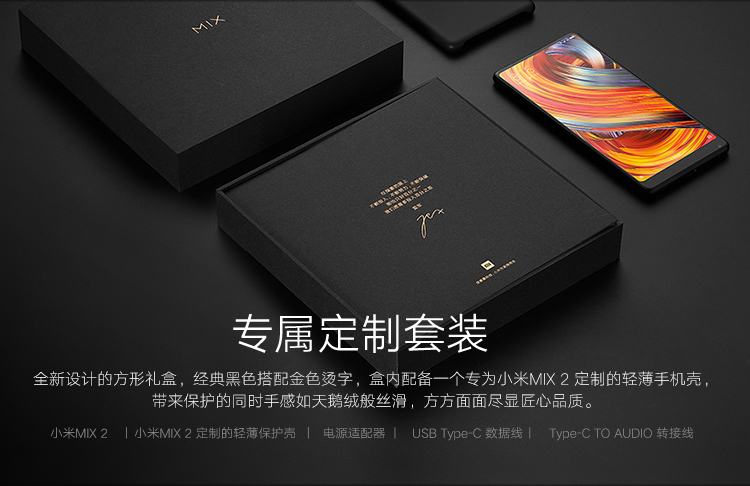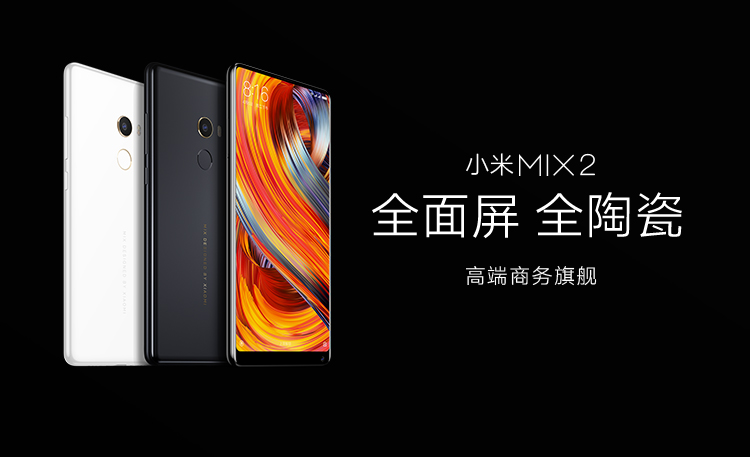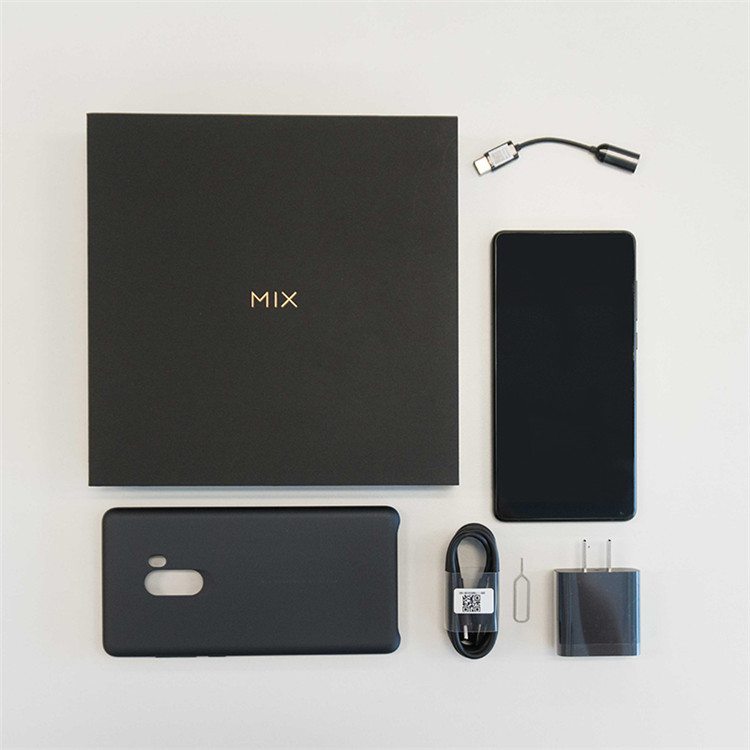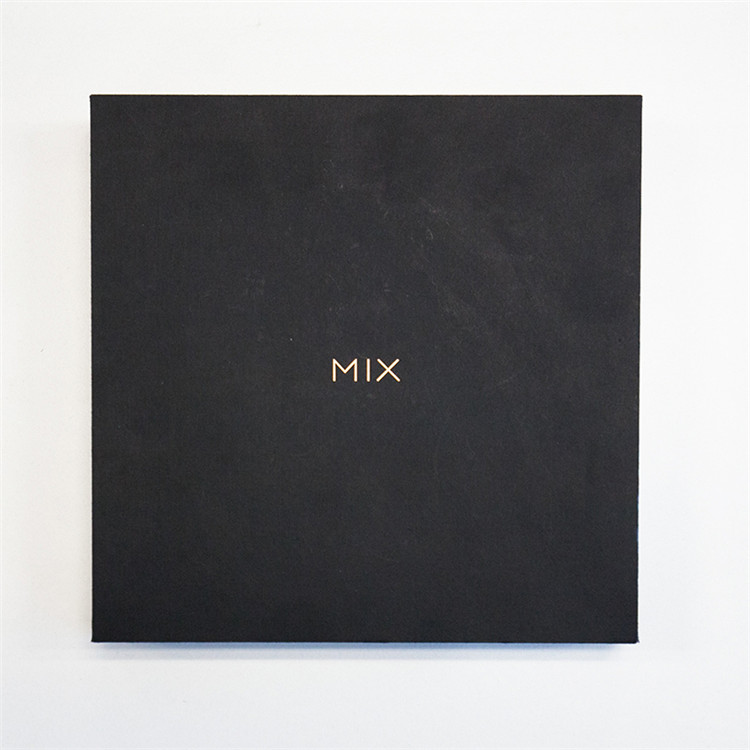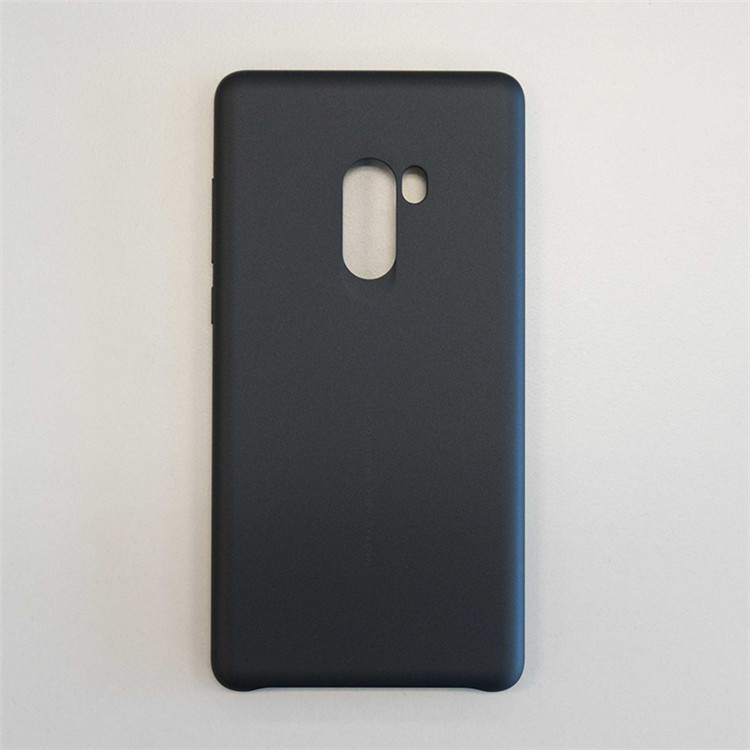.